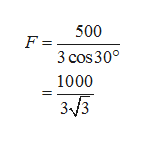A 500-lb load hangs from three cables of equal length that are anchored at the points (-2,0,0), (1,31/2,0), and (1,-31/2,0). The load is located at (0,0,-2*151/2). Find the vectors describing the forces on the cables due to the load. What is the vector describing the force on the cable anchored at (-2,0,0)?

Question

A 500-lb load hangs from three cables of equal length that are anchored at the points (-2,0,0), (1,31/2,0), and (1,-31/2,0). The load is located at (0,0,-2*151/2). Find the vectors describing the forces on the cables due to the load. What is the vector describing the force on the cable anchored at (-2,0,0)?

Step 1

It is given that a load of 500-lb is hanged from three cables of equal lengths that are anchored at the points say, A, B and C respectively as shown.

Step 2

The point at which the load is located say D is,

Step 3

Note that, each cable is holding a weight of one third of 500 lb making an angle of 30 degree with the...help_outlineImage Transcriptionclose500 F 3 cos 30 1000 33 fullscreen

Want to see the full answer?

See Solution

Want to see this answer and more?

Our solutions are written by experts, many with advanced degrees, and available 24/7

See Solution
Tagged in

Calculus